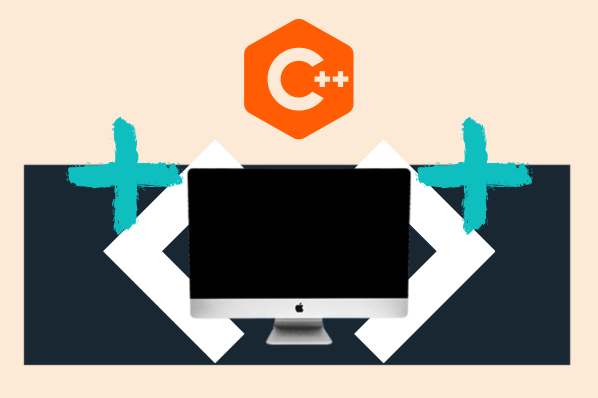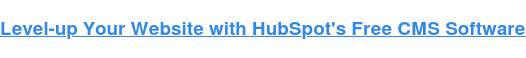# C++ Functions List: 20 Definitions & ExamplesPublished:

C++ functions are indispensable for developing powerful applications. They're versatile, flexible, and can be used to accomplish everything from building a web page to designing a video game interface.But, here's the catch. C++ functions aren't as straightforward as Python functions or SQL functions. There's a little more that goes into their syntax that makes them unique from other programming languages.

In this post, we'll explore a list of the most common functions used in C++. We'll define what each one does, then provide a use-case example for when you might use this code. Keep this guide handy as you can use it as a reference point for your C++ programming needs.## List of C++ Functions

### 1. Print: std::cout

This is the standard output stream object used for printing to the console.

Example:

`#include <iostream>int main() {  std::cout << "Hello, World!" << std::endl;  return 0;}`

Output:

Hello, World!

### 2. Maximum: std::max

The maximum function returns the larger of two numbers.

Example:

`#include <iostream>#include <algorithm>int main() {  int a = 5;  int b = 8;  int maxVal = std::max(a, b);  std::cout << "The maximum value is " << maxVal << std::endl;  return 0;}`

Output:

The maximum value is 8

### 3. Minimum: std::max

The minimum function returns the smaller of two numbers.

Example:

`#include <iostream>#include <algorithm>int main() {  int a = 5;  int b = 8;  int minVal = std::min(a, b);  std::cout << "The minimum value is " << minVal << std::endl;  return 0;}`

Output:

The minimum value is 5

### 4. Uppercase: std::toupper

The uppercase function converts a character to uppercase.

Example:

`#include <iostream>#include <cctype>int main() {  char ch = 'a';  char uppercaseCh = std::toupper(ch);  std::cout << "The uppercase character is " << uppercaseCh << std::endl;  return 0;}`

Output:

The uppercase character is A

### 5. Input: std::cin

This function creates the standard object used for reading input from the console.

Example:

`#include <iostream>int main() {  std::cout << "Enter a number: ";  int num;  std::cin >> num;  std::cout << "You entered " << num << std::endl;  return 0;}`

Output:

Enter a number: 10

You entered 10

### 6. New Line: std::end1

This function inserts a new line and flushes the output stream.

Example:

`#include <iostream>int main() {  std::cout << "Line 1" << std::endl;  std::cout << "Line 2" << std::endl;  return 0;}`

Output:

Line 1

Line 2

### 7. Vector: std::vector

This is a class template that represents a dynamic array and provides various functions for element access and manipulation.

Example:

`#include <iostream>#include <vector>int main() {  std::vector<int> numbers = {1, 2, 3, 4, 5};  int firstElement = numbers;  std::cout << "The first element is " << firstElement << std::endl;  return 0;}`

Output:

The first element is 1

### 8. Sort: std::sort

The sort function organizes the elements of a container in ascending order.

Example:

`#include <iostream>#include <vector>#include <algorithm>int main() {  std::vector<int> numbers = {5, 2, 8, 1, 3};  std::sort(numbers.begin(), numbers.end());  for (int i = 0; i < numbers.size(); i++) {    std::cout << numbers[i] << " ";  }std::cout << std::endl;  return 0;}`

Output:

1 2 3 5 8

### 9. Find: std::find

The find function searches for a value in a container and returns an iterator to the first occurrence.

Example:

`#include <iostream>#include <vector>#include <algorithm>int main() {  std::vector<int> numbers = {1, 2, 3, 4, 5};  auto it = std::find(numbers.begin(), numbers.end(), 3);  if (it != numbers.end()) {    std::cout << "The element 3 is found at index " << it - numbers.begin() << std::endl;  } else {    std::cout << "The element 3 is not found" << std::endl;  }  return 0;}`

Output:

The element 3 is found at index 2

### 10. Get Line: std::getline

The get line function reads a line of text from an input stream.

Example:

`#include <iostream>#include <string>int main() {  std::string line;  std::getline(std::cin, line);  std::cout << "The line you entered is: " << line << std::endl;     return 0;}`

Output:

This code will prompt the user to enter a line of text and then print the line back to the user.

### 11. Random Number Generation: std::rand

This function generates a pseudo-random integer within a specified range.

Example:

`#include <iostream>#include <random>int main() {  std::random_device rd;  std::mt19937 gen(rd());  std::uniform_int_distribution<> dis(0, 99);    int randomNum = dis(gen);  std::cout << "The random number is: " << randomNum << std::endl;  return 0;}`

Output:

This code will generate a random number between 0 and 99.

### 12. C++ Recursion

A recursive function is a function that solves a problem by solving smaller instances of the same problem. It does so by breaking up larger problems into smaller, subproblems.

Example:

`#include <iostream>int factorial(int n) {    // Base case: factorial of 0 is 1    if (n == 0) {        return 1;    }    // Recursive call: factorial(n) = n * factorial(n-1)    return n * factorial(n - 1);}int main() {    int num = 5;    int result = factorial(num);    std::cout << "Factorial of " << num << " is " << result << std::endl;    return 0;}`

Output:

Factorial of 5 is 120

Function overloading allows you to define multiple functions with the same name but using different parameter lists. With this technique, you can create functions that perform similar tasks but can handle different types of inputs or different numbers of parameters. The compiler determines which function to call based on the arguments passed during the function call.

Example:

`#include <iostream>void print(int num) {    std::cout << "Printing an integer: " << num << std::endl;}void print(double num) {    std::cout << "Printing a double: " << num << std::endl;}void print(const char* str) {    std::cout << "Printing a string: " << str << std::endl;}int main() {    print(10);            // Calls print(int)    print(3.14);          // Calls print(double) std::endl;    print("Hello World"); // Calls print(const char*)    return 0;}`

Output:

Printing an integer: 10

Printing a double: 3.14

Printing a string: Hello World

### 14. Pass a File to a Function: std::ifstream

To pass a file to a function, you can use the file stream classes provided by the standard library.

Example:

`#include <iostream>#include <fstream>void processFile(std::ifstream& file) {    // Function code to process the file    std::string line;    while (std::getline(file, line)) {        std::cout << line << std::endl;    }}int main() {    std::ifstream inputFile("filename.txt");    if (inputFile.is_open()) {        processFile(inputFile);        inputFile.close();    } else {        std::cout << "Failed to open the file." << std::endl;    }    return 0;}`

Output:

This function would open your file.

### 15. Array Length Function

You can determine the length of an array by using the sizeof operator and dividing it by the size of an individual array element.

Example:

`#include <iostream>int main() {    int array[] = {1, 2, 3, 4, 5};    int length = sizeof(array) / sizeof(array);    std::cout << "Length of the array: " << length << std::endl;        std::cout << line << std::endl;    return 0;}`

Output:

Length of the array: 5

### 16. Return a String From a Function: std::string

To return a string from a function, you can use the std::string class provided by the C++ Standard Library.

Example:

`#include <iostream>#include <string>std::string getString() {    std::string message = "Hello, World!";    return message;}int main() {    std::string result = getString();    std::cout << result << std::endl;    return 0;}`

Output:

Hello, World!

### 17. Boolean Expressions: bool

A boolean expression is an expression that evaluates to a boolean value (true or false). It typically involves the use of relational and logical operators to compare values or combine multiple boolean expressions.

Example:

`#include <iostream>int main() {    int x = 5;    int y = 8;    bool result;    result = (x == y);  // false, because 5 is not equal to 8    result = (x > 2 && y < 10);  // true, because x is greater than 2 and y is less than 10    result = (x <= 3 || y >= 5);  // true, because either x is less than or equal to 3 or y is greater than or equal to 5    result = !(x == 5);  // false, because x is equal to 5, but the logical NOT operator negates the result    return 0;}`

Output:

False

### 18. Array Sorting: std::array::sort

This function sorts the elements of a fixed-size array in ascending order.

Example:

`#include <iostream>#include <array>#include <algorithm>int main() {  std::array<int, 5> numbers = {5, 2, 8, 1, 3};  std::sort(numbers.begin(), numbers.end());  for (int i = 0; i < numbers.size(); i++) {    std::cout << numbers[i] << " ";  }  std::cout << std::endl;  return 0;}`

Output:

1 2 3 5 8

### 19. Array Copying: std::copy

The copy function copies elements from one array to another.

Example:

`#include <iostream>#include <algorithm>int main() {  int source[] = {1, 2, 3, 4, 5};  std::sort(numbers.begin(), numbers.end());  for (int i = 0; i < 5; i++) {    std::cout << destination[i] << " ";  }  std::cout << std::endl;  return 0;}`

Output:

1 2 3 4 5

### 20. Array Reverse: std::reverse

This function reverses the order of elements in an array.

Example:

`#include <iostream>#include <algorithm>int main() {  int numbers[] = {1, 2, 3, 4, 5};  std::reverse(numbers, numbers + 5);  for (int i = 0; i < 5; i++) {    std::cout << numbers[i] << " ";  }  std::cout << std::endl;  return 0;}`

Output:

5 4 3 2 1

## Using Functions in C++

These functions will help you create more efficient and powerful code, empowering you to scale projects and take your programming to the next level.

Remember to continuously practice and experiment as that will help strengthen your understanding and build on your abilities. Lastly, bookmark this guide so you can return to it whenever you need to brush up on an under-utilized function or discover new functions that you might not have worked with before.

Author's Note: This post was written/edited by a human with the assistance of generative AI.## Related Articles

A free suite of content management tools for marketers and developers.